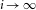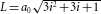## Main

In two dimensions, a saddle point in the electronic band structure leads to a divergence in the density of states, also known as a Van Hove singularity1 (VHS). When the Fermi energy (EF) is close to the VHS, interactions, however weak, are magnified by the enhanced density of states (DOS), resulting in instabilities, which can give rise to new phases of matter2,3,4,5 with desirable properties. This implies the possibility of engineering material properties by bringing EF and the VHS together. However, in most materials one cannot change the position of the VHS in the band structure. Instead, it may be possible to tune EF through the VHS by chemical doping8 or by gating7. In this regard, graphene, the recently discovered two-dimensional form of carbon, is quite special5,9. It has linearly dispersing bands at the K (K′) point in the Brillouin zone, the so-called Dirac points, and a DOS that is linear and vanishes at Dirac point. The fact that this material is truly two-dimensional and has a low DOS means that it cannot screen applied electric fields, allowing for strong gating and ambipolar behaviour7. However, although the band structure of graphene5 contains a VHS, its large distance from the Dirac point makes it prohibitively difficult to reach by either gating or chemical doping. We show that by introducing a rotation between stacked graphene layers, it is possible to induce VHSs that are within the range of EF achievable by gate tuning. As the samples studied here are not intentionally doped, EF is within a few millielectronvolts of the Dirac point.

Rotation between graphene layers is often observed as a Moiré pattern on graphite surfaces10, as illustrated in Fig. 1. Graphite consists of stacked layers of graphene, the lattice structure of which contains two interpenetrating triangular sublattices, denoted A and B. In the most common (Bernal) stacking, adjacent layers are shifted by one atomic spacing, so that B atoms of layer 2 (B2) sit directly on top of A atoms of layer 1 (A1) and B1 and A2 atoms are in the centre of the hexagons of the opposing layer. Assuming a rotation through an angle θ about an A1 (B2) site in bilayer graphene, a set of conditions for commensurate periodic structures leading to Moiré patterns is easily derived11 cos(θi)=(3i2+3i+1/2)/(3i2+3i+1), with i being an integer (i=0, θ=60 corresponds to AA stacking and, θ=0 to AB stacking) and lattice constant of the superlattice, where a02.46 Å is the atomic lattice constant. For the extended Moiré pattern in Fig. 1θi=1.79 is obtained from the superlattice period, L=7.7±0.3 nm, corresponding to i=18.Figure 1: STM of a graphene flake on a freshly cleaved surface of highly oriented pyrolitic graphite revealing a Moiré pattern.

Thus far, most work on Moiré patterns focused on structural aspects revealed by scanning tunnelling microscopy (STM), but their effect on the electronic properties has not been addressed. By using scanning tunnelling spectroscopy (STS), we find that rotation markedly alters the DOS. Figure 2 shows the tunnelling differential conductance, dI/dV, a quantity proportional to the local DOS (ref. 12). In regions inside the Moiré pattern (M1 and M2), the spectra develop two sharp peaks flanking the Dirac points with energy separation ΔEvhs82 meV. Below, we show that these peaks correspond to rotation-induced VHSs. The effect of the VHS on the DOS is evident in the energy dependence of the dI/dV maps. Close to the VHS, the maps develop a strong density modulation (Figs 2f and 3c), characteristic of charge-density waves (CDWs), suggesting an impending Fermi-surface instability. In contrast, for energies away from the VHS, the charge density becomes nearly homogeneous.Figure 2: STS inside and outside a Moiré pattern with θ=1.79∘.

To explore the angle dependence of ΔEvhs, we studied graphene layers prepared by chemical vapour deposition13 (CVD). CVD graphene layers have a strong twisting tendency revealed by Moiré patterns with a range of rotation angles. The example in Fig. 3a shows a pattern, corresponding to θ1.16. The STS spectra in this region reveal strong VHSs (Fig. 3b) with a much smaller ΔEvhs12 meV. The pronounced spatial modulation of the dI/dV maps at energies close to these VHSs (Fig. 3c), indicating the formation of a CDW, is significantly stronger than for the pattern in Fig. 2f, where the more widely separated VHSs are farther away from the Fermi energy. For a pattern with an even larger angle, θ3.4, and ΔEvhs430 meV, the localization is weaker still (see Supplementary Fig. S3), consistent with theoretical predictions14.

We next show that the VHSs are induced by the rotation and use the model developed in ref. 11 to derive the angle dependence of ΔEvhs. A rotation between two graphene layers causes a shift between the corresponding Dirac points in momentum space, so that the Dirac wavevector of the rotated layer is Kθ=KK, where ΔK=K×2sin(θ/2). If we use the same origin of momentum for the two layers, so that a uniform hopping couples states of the same momentum in both layers, the zero-energy states do not occur at k=0, but rather at k=−ΔK/2 in layer 1 and kK/2 in layer 2. Unlike in the AB stacked bilayer, there is no direct coupling of the zero-energy states of one layer to the zero-energy states of the other. As shown in ref. 11, the states near the Dirac cone of each layer couple with amplitudes of order tθ≈0.4 t to states of energy ±vFΔK of the opposing layer and the linear dispersion is preserved near zero energy. Here, t is the interlayer hopping for unrotated layers and vF106 m s−1 is the Fermi velocity. The two Dirac cones intersect near the centre of the superlattice Brillouin zone and hybridize, resulting in a saddle point in the energy dispersion and in two symmetric VHSs (Fig. 4a).